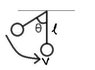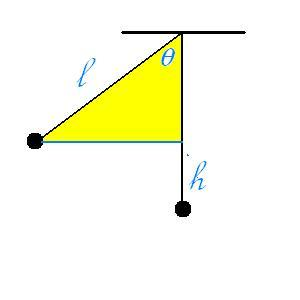# Velocity of the pendulum bob

• ubergewehr273

## Homework Statement

Show that a simple pendulum bob which has been pulled aside from its equilibrium position through an angle ##\theta## and then released will pass through the equilibrium position with speed ##v = \sqrt{2gl(1-cos\theta)}##, where ##l## is the length of the pendulum.

## Homework Equations

##K.E = \frac{1}{2}mv^2##
##P.E = mgh##

## The Attempt at a Solution

I tried a lot in finding the start of the solution but I have no idea from where to start.

Hi Ashes. You have to use conservation of energy here. Try it.

Draw a picture first.

Here is the diagram.

#### Attachments

•Pendulum.jpg
4.6 KB · Views: 851
Here is the diagram.

The diagram is correct. Try to apply law of conservation of energy. What are the values of initial and final kinetic and potential energies?

You may find some ambiguity of the term
L(1-cos theta)

But this term is the horizontal distance from the equilibrium position of the bob to any position the bob can occupy .

:)

No, it is not the horizontal distance. What is the hight of the bob above the deepest position, when it deflects by angle theta from the vertical? See picture. Find h .Oh sorry ,,
it is the height though..

No, it is not the horizontal distance. What is the hight of the bob above the deepest position, when it deflects by angle theta from the vertical? See picture. Find h .
I got it!
##cos\theta=\frac{l-h}{l}##
##\Rightarrow l-h=lcos\theta##
##\Rightarrow h=l(1-cos\theta)##

Since, the pendulum starts moving from the non - equilibrium position, its initial velocity would be zero.
So, the final velocity ##v## is given by,
##v=\sqrt{2gh}## where ##g## is acceleration due to gravity.
Also, the maximum velocity is achieved at the equilibrium position.
So, substituting for ##h## we get,
##v=\sqrt{2gl(1-cos\theta)}##
Thanks a lot ehild.

Last edited:
So, substituting for ##h## we get,
##v=\sqrt{2gl(l-cos\theta)}##
Thanks a lot ehild.
There is a typo in the final formula. It has to be ##v=\sqrt{2gl(1-cos\theta)}##

Anyway, you understood and you did it, congrats! :)

Thanks a lot for the clue ehild.
P.S I got the typo fixed.